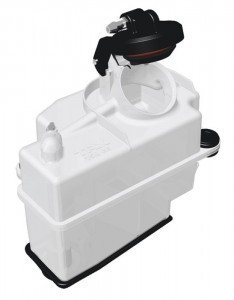# Fuel Tank Dimensions

The fuel tank capacity depends on the size of the tank.The 500 and 1,000 gallon tanks are usually underground tanks, while the 275 and 330 gallons are above ground. A 275 is about 26" X 44" X 5 ft long.

### Measuring a 275 H Gallon Fuel Tank (26” diameter)

1” is equal to 6 gallons, while 2 in is equal to 14 inches; 3 inches is equal to 23 gallons. 4 gallons is equal to 33 and 5 inches to 43 gal. 6 in is equivalent to 54; 7 inches is equal to 65 gal; 8 inches is the same as 77 gallons and 9 inches is equal to 89 gallons.

10 inches is equal to 100 gal; 12 in is 125 gal; 14” is 149 gal; 16 in is equivalent to 174 and 18”, 197 gal. When checking the fuel tank capacity, bear in mind that 20 in is the same as 221 gal; 22 in is equal to 241 gal. 24” is about 260 gallons and 26” in is 275 gal.

### Measuring a 275 V Gallon Fuel Tank (26” diameter)

1 inch is equal to 2 gallons and 2 in about 5 gal. Size 3 is equal to 9 gal; size 4 is 14 gal and 5 inches 20 gallons. The other measurements are 6 in (26 gal), 8 in (39 gal), 10 in (53 gal), 12” (67 gal), 14” (82 gal), 16 in (97 gal), 18 in (112 gal) and 20 in (127 gallons).

If the fuel tank capacity is at 22 in, that is about 142 gallons. Size 24 is equal to 157 gallons; 26” is equal to 172 gallons.

The other inch to gallon equivalents are the following. 29 in (195 gal), 30 in (203 gal), 32 in (217 gal), 34” (231 gal) and 36 inches about 244 gallons. 38 inches is equal to 256 gallons; 40 inches is equal to 265 gallons. 41 inches is about 268 gallons and 42 inches equal to 271 gallons.

### Measuring a 330 Gallon Fuel Tank (44.5” diameter)

1 inch is equal to 3 gallons; 3 inches is equal to 11 gallons and 5 inches 23 gallons; 7 inches of fuel translates to 37 gallons and 9 inches, 52 gallons. If the fuel tank capacity is at 13 inches, it is 85 gallons; 15 in is equal to 102 gallons and 17 inches, 119 gallons. 19 inches translates to 136 gallons while 21 inches is about 152 gallons.

Other measurements: 23 inches (169 gal), 25 inches (186 gal), 27 gal (203 gal) and 29 in (220 gal). If it is 31 inches, that is about 237 gallons; if it is 3 inches, the gallons number 253.

If the fuel is 35 inches that is equal to 270 gallons; 36 inches, 278; 37 inches, 285 gallons and 39 inches, 299 gallons. 306 gallons is about 40 inches and 317 gallons, 42 inches.

The fuel tank capacity given here are only approximate measures. Manufacturers may employ different designs that can change its capacity.